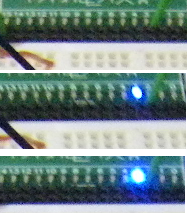The project has been updated to include a LED dimmer as a PWM example.

The additional code included in the updated project source on Codeplex follows:

## UI: XAML

In MainPage.xaml:

Add two more rows to the <grid> element:

<RowDefinition Height="Auto"/>

<RowDefinition Height="60"/>

Then add a TextBlock and Slider for those rows:

<TextBlock x:Name="textBlock" HorizontalAlignment="Left" Height="34" Margin="10,10.333,0,-44.333" Grid.Row="9" TextWrapping="Wrap" Text="PWM: LED Dimmer" VerticalAlignment="Top" Width="273"/>

<Slider x:Name="slider" HorizontalAlignment="Left" Height="47" Margin="0,34.333,0,-81.333" Grid.Row="10" VerticalAlignment="Center" Width="350" ValueChanged="slider_ValueChanged" Maximum="255" SmallChange="5" StepFrequency="5"/>

# Define Pin 10 for the Dimmer (PWM)

In MainPage.cs in the class declarations:

//Dimmer (Use Pin 10):

private const int PWM_PIN = 10;

## Set Pin 10 to PWM Mode

In OnConnected()

//Dimmer:

arduino.pinMode(PWM_PIN, PinMode.PWM);

# Add Slider Event Handler to update PWM value:

PWM value range from 0 to 255. In the Slider's XAML, the max value is set to 255.
At the bottom of the class insert:

private void slider_ValueChanged(object sender, RangeBaseValueChangedEventArgs e)

{

byte val = (byte) slider.Value;

arduino.analogWrite(PWM_PIN, val);

}

## Hardware

Connect Pin 10 to a LED as per Blinky.

AND AWAY YOU GO.  As you slide the slider the intensity of the LED will vary.